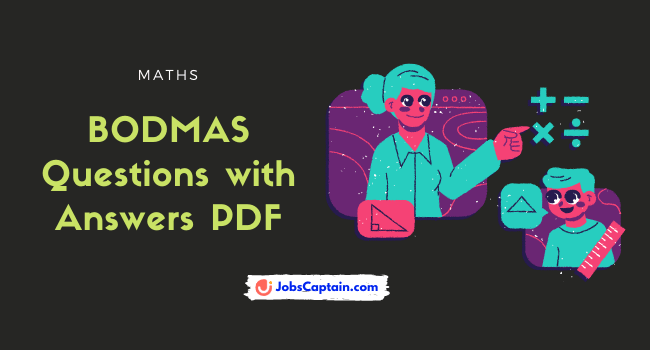By | October 1, 2022BODMAS is a method used to solve mathematical problems. The acronym stands for Brackets, Orders, Division, Multiplication, Addition, and Subtraction. In this blog post, we will provide BODMAS questions and answers to help you better understand this concept.

Download BODMAS Question PDF for RRB Group D Exam preparation. In this PDF set, we cover important questions and answers with solutions. However, BODMAS questions are also helpful in every competitive exam like SSC, UPSC, RRB and many more.

BODMAS Rule
BBracket (Brackets are solved in order of (), {} and [] respectively.
OOf Þ multiplication, but it is to be done before division.
DDivision
MMultiplication
SSubtraction

Question 1: The value of  20 × (10 – 6) ÷ 5 = _______________ is.

(A) 20

(B) 16

(C) 17

(D) 19

(B) 16

Explanation:

20 × (10 – 6) ÷ 5
= 20 × 4 ÷ 5
= 80 ÷ 5
= 16

Question 2: The value of 14 ÷ { ( 5 of 2 – 3 ) } × 4 (7 − 2) is :

(A) 44

(B) 10

(C) 1/10

(D)  14/19

(A) 44

Explanation:

14 ÷ { ( 5 of 2 – 3 ) } × 4 (7 − 2)
= 14 ÷ 7 ×4 (5)
= 2 × 4 (5)
= 2 × 20
= 40